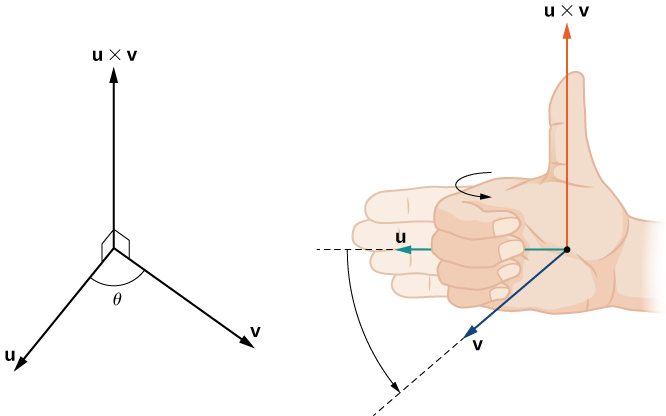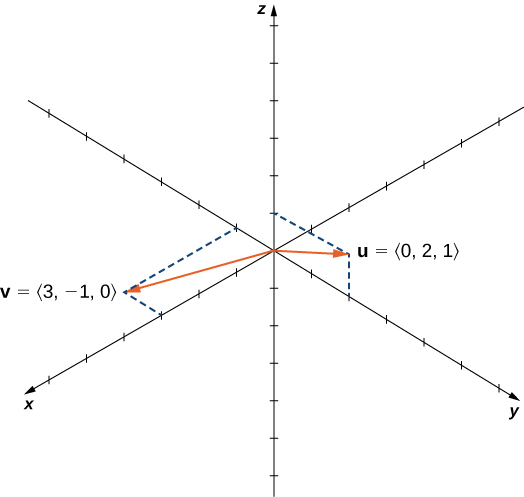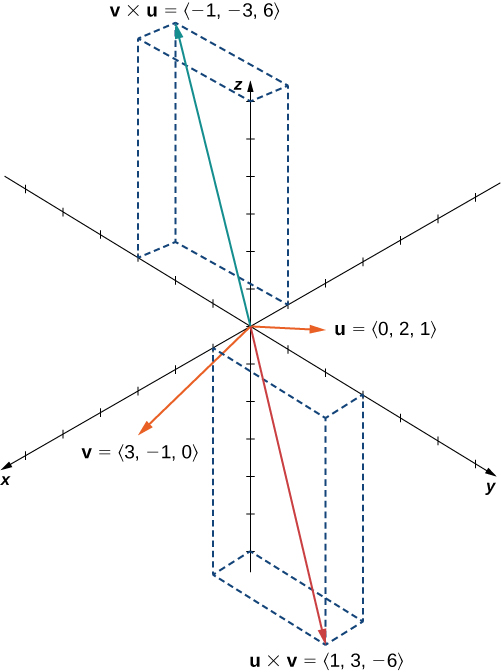# 2.4 The cross product  (Page 2/16)

 Page 2 / 16The direction of u × v is determined by the right-hand rule.

Notice what this means for the direction of $\text{v}\phantom{\rule{0.2em}{0ex}}×\phantom{\rule{0.2em}{0ex}}\text{u}.$ If we apply the right-hand rule to $\text{v}\phantom{\rule{0.2em}{0ex}}×\phantom{\rule{0.2em}{0ex}}\text{u},$ we start with our fingers pointed in the direction of $\text{v},$ then curl our fingers toward the vector $\text{u}.$ In this case, the thumb points in the opposite direction of $\text{u}\phantom{\rule{0.2em}{0ex}}×\phantom{\rule{0.2em}{0ex}}\text{v}.$ (Try it!)

## Anticommutativity of the cross product

Let $\text{u}=⟨0,2,1⟩$ and $\text{v}=⟨3,-1,0⟩.$ Calculate $\text{u}\phantom{\rule{0.2em}{0ex}}×\phantom{\rule{0.2em}{0ex}}\text{v}$ and $\text{v}\phantom{\rule{0.2em}{0ex}}×\phantom{\rule{0.2em}{0ex}}\text{u}$ and graph them.Are the cross products u × v and v × u in the same direction?

We have

$\begin{array}{ccc}\hfill \text{u}\phantom{\rule{0.2em}{0ex}}×\phantom{\rule{0.2em}{0ex}}\text{v}& =\hfill & ⟨\left(0+1\right),\text{−}\left(0-3\right),\left(0-6\right)⟩=⟨1,3,-6⟩\hfill \\ \hfill \text{v}\phantom{\rule{0.2em}{0ex}}×\phantom{\rule{0.2em}{0ex}}\text{u}& =\hfill & ⟨\left(-1-0\right),\text{−}\left(3-0\right),\left(6-0\right)⟩=⟨-1,-3,6⟩.\hfill \end{array}$

We see that, in this case, $\text{u}\phantom{\rule{0.2em}{0ex}}×\phantom{\rule{0.2em}{0ex}}\text{v}=\text{−}\left(\text{v}\phantom{\rule{0.2em}{0ex}}×\phantom{\rule{0.2em}{0ex}}\text{u}\right)$ ( [link] ). We prove this in general later in this section.The cross products u × v and v × u are both orthogonal to u and v , but in opposite directions.

Suppose vectors $\text{u}$ and $\text{v}$ lie in the xy -plane (the z -component of each vector is zero). Now suppose the x - and y -components of $\text{u}$ and the y -component of $\text{v}$ are all positive, whereas the x -component of $\text{v}$ is negative. Assuming the coordinate axes are oriented in the usual positions, in which direction does $\text{u}\phantom{\rule{0.2em}{0ex}}×\phantom{\rule{0.2em}{0ex}}\text{v}$ point?

Up (the positive z -direction)

The cross products of the standard unit vectors $\text{i},\text{j},$ and $\text{k}$ can be useful for simplifying some calculations, so let’s consider these cross products. A straightforward application of the definition shows that

$\text{i}\phantom{\rule{0.2em}{0ex}}×\phantom{\rule{0.2em}{0ex}}\text{i}=\text{j}\phantom{\rule{0.2em}{0ex}}×\phantom{\rule{0.2em}{0ex}}\text{j}=\text{k}\phantom{\rule{0.2em}{0ex}}×\phantom{\rule{0.2em}{0ex}}\text{k}=0.$

(The cross product of two vectors is a vector, so each of these products results in the zero vector, not the scalar $0.\right)$ It’s up to you to verify the calculations on your own.

Furthermore, because the cross product of two vectors is orthogonal to each of these vectors, we know that the cross product of $\text{i}$ and $\text{j}$ is parallel to $\text{k}.$ Similarly, the vector product of $\text{i}$ and $\text{k}$ is parallel to $\text{j},$ and the vector product of $\text{j}$ and $\text{k}$ is parallel to $\text{i}.$ We can use the right-hand rule to determine the direction of each product. Then we have

$\begin{array}{cccccccc}\hfill \text{i}\phantom{\rule{0.2em}{0ex}}×\phantom{\rule{0.2em}{0ex}}\text{j}& =\hfill & \text{k}\hfill & & & \hfill \text{j}\phantom{\rule{0.2em}{0ex}}×\phantom{\rule{0.2em}{0ex}}\text{i}& =\hfill & \text{−}\text{k}\hfill \\ \hfill \text{j}\phantom{\rule{0.2em}{0ex}}×\phantom{\rule{0.2em}{0ex}}\text{k}& =\hfill & \text{i}\hfill & & & \hfill \text{k}\phantom{\rule{0.2em}{0ex}}×\phantom{\rule{0.2em}{0ex}}\text{j}& =\hfill & \text{−}\text{i}\hfill \\ \hfill \text{k}\phantom{\rule{0.2em}{0ex}}×\phantom{\rule{0.2em}{0ex}}\text{i}& =\hfill & \text{j}\hfill & & & \hfill \text{i}\phantom{\rule{0.2em}{0ex}}×\phantom{\rule{0.2em}{0ex}}\text{k}& =\hfill & \text{−}\text{j}.\hfill \end{array}$

These formulas come in handy later.

## Cross product of standard unit vectors

Find $\text{i}\phantom{\rule{0.2em}{0ex}}×\phantom{\rule{0.2em}{0ex}}\left(\text{j}\phantom{\rule{0.2em}{0ex}}×\phantom{\rule{0.2em}{0ex}}\text{k}\right).$

We know that $\text{j}\phantom{\rule{0.2em}{0ex}}×\phantom{\rule{0.2em}{0ex}}\text{k}=\text{i}.$ Therefore, $\text{i}\phantom{\rule{0.2em}{0ex}}×\phantom{\rule{0.2em}{0ex}}\left(\text{j}\phantom{\rule{0.2em}{0ex}}×\phantom{\rule{0.2em}{0ex}}\text{k}\right)=\text{i}\phantom{\rule{0.2em}{0ex}}×\phantom{\rule{0.2em}{0ex}}\text{i}=0.$

Find $\left(\text{i}\phantom{\rule{0.2em}{0ex}}×\phantom{\rule{0.2em}{0ex}}\text{j}\right)\phantom{\rule{0.2em}{0ex}}×\phantom{\rule{0.2em}{0ex}}\left(\text{k}\phantom{\rule{0.2em}{0ex}}×\phantom{\rule{0.2em}{0ex}}\text{i}\right).$

$\text{−}\text{i}$

As we have seen, the dot product is often called the scalar product because it results in a scalar. The cross product results in a vector, so it is sometimes called the vector product    . These operations are both versions of vector multiplication, but they have very different properties and applications. Let’s explore some properties of the cross product. We prove only a few of them. Proofs of the other properties are left as exercises.

## Properties of the cross product

Let $\text{u},\text{v},$ and $\text{w}$ be vectors in space, and let $c$ be a scalar.

$\begin{array}{cccccccc}\text{i.}\hfill & & & \hfill \text{u}\phantom{\rule{0.2em}{0ex}}×\phantom{\rule{0.2em}{0ex}}\text{v}& =\hfill & \text{−}\left(\text{v}\phantom{\rule{0.2em}{0ex}}×\phantom{\rule{0.2em}{0ex}}\text{u}\right)\hfill & & \text{Anticommutative property}\hfill \\ \text{ii.}\hfill & & & \hfill \text{u}\phantom{\rule{0.2em}{0ex}}×\phantom{\rule{0.2em}{0ex}}\left(\text{v}+\text{w}\right)& =\hfill & \text{u}\phantom{\rule{0.2em}{0ex}}×\phantom{\rule{0.2em}{0ex}}\text{v}+\text{u}\phantom{\rule{0.2em}{0ex}}×\phantom{\rule{0.2em}{0ex}}\text{w}\hfill & & \text{Distributive property}\hfill \\ \text{iii.}\hfill & & & \hfill c\left(\text{u}\phantom{\rule{0.2em}{0ex}}×\phantom{\rule{0.2em}{0ex}}\text{v}\right)& =\hfill & \left(c\text{u}\right)\phantom{\rule{0.2em}{0ex}}×\phantom{\rule{0.2em}{0ex}}\text{v}=\text{u}\phantom{\rule{0.2em}{0ex}}×\phantom{\rule{0.2em}{0ex}}\left(c\text{v}\right)\hfill & & \text{Multiplication by a constant}\hfill \\ \text{iv.}\hfill & & & \hfill \text{u}\phantom{\rule{0.2em}{0ex}}×\phantom{\rule{0.2em}{0ex}}0& =\hfill & 0\phantom{\rule{0.2em}{0ex}}×\phantom{\rule{0.2em}{0ex}}\text{u}=0\hfill & & \text{Cross product of the zero vector}\hfill \\ \text{v.}\hfill & & & \hfill \text{v}\phantom{\rule{0.2em}{0ex}}×\phantom{\rule{0.2em}{0ex}}\text{v}& =\hfill & 0\hfill & & \text{Cross product of a vector with itself}\hfill \\ \text{vi.}\hfill & & & \hfill \text{u}·\left(\text{v}\phantom{\rule{0.2em}{0ex}}×\phantom{\rule{0.2em}{0ex}}\text{w}\right)& =\hfill & \left(\text{u}\phantom{\rule{0.2em}{0ex}}×\phantom{\rule{0.2em}{0ex}}\text{v}\right)·\text{w}\hfill & & \text{Scalar triple product}\hfill \end{array}$

## Proof

For property $\text{i}.,$ we want to show $\text{u}\phantom{\rule{0.2em}{0ex}}×\phantom{\rule{0.2em}{0ex}}\text{v}=\text{−}\left(\text{v}\phantom{\rule{0.2em}{0ex}}×\phantom{\rule{0.2em}{0ex}}\text{u}\right).$ We have

$\begin{array}{cc}\hfill \text{u}\phantom{\rule{0.2em}{0ex}}×\phantom{\rule{0.2em}{0ex}}\text{v}& =⟨{u}_{1},{u}_{2},{u}_{3}⟩\phantom{\rule{0.2em}{0ex}}×\phantom{\rule{0.2em}{0ex}}⟨{v}_{1},{v}_{2},{v}_{3}⟩\hfill \\ & =⟨{u}_{2}{v}_{3}-{u}_{3}{v}_{2},\text{−}{u}_{1}{v}_{3}+{u}_{3}{v}_{1},{u}_{1}{v}_{2}-{u}_{2}{v}_{1}⟩\hfill \\ & =\text{−}⟨{u}_{3}{v}_{2}-{u}_{2}{v}_{3},\text{−}{u}_{3}{v}_{1}+{u}_{1}{v}_{3},{u}_{2}{v}_{1}-{u}_{1}{v}_{2}⟩\hfill \\ & =\text{−}⟨{v}_{1},{v}_{2},{v}_{3}⟩\phantom{\rule{0.2em}{0ex}}×\phantom{\rule{0.2em}{0ex}}⟨{u}_{1},{u}_{2},{u}_{3}⟩\hfill \\ & =\text{−}\left(\text{v}\phantom{\rule{0.2em}{0ex}}×\phantom{\rule{0.2em}{0ex}}\text{u}\right).\hfill \end{array}$

Unlike most operations we’ve seen, the cross product is not commutative. This makes sense if we think about the right-hand rule.

where we get a research paper on Nano chemistry....?
what are the products of Nano chemistry?
There are lots of products of nano chemistry... Like nano coatings.....carbon fiber.. And lots of others..
learn
Even nanotechnology is pretty much all about chemistry... Its the chemistry on quantum or atomic level
learn
da
no nanotechnology is also a part of physics and maths it requires angle formulas and some pressure regarding concepts
Bhagvanji
Preparation and Applications of Nanomaterial for Drug Delivery
revolt
da
Application of nanotechnology in medicine
what is variations in raman spectra for nanomaterials
I only see partial conversation and what's the question here!
what about nanotechnology for water purification
please someone correct me if I'm wrong but I think one can use nanoparticles, specially silver nanoparticles for water treatment.
Damian
yes that's correct
Professor
I think
Professor
Nasa has use it in the 60's, copper as water purification in the moon travel.
Alexandre
nanocopper obvius
Alexandre
what is the stm
is there industrial application of fullrenes. What is the method to prepare fullrene on large scale.?
Rafiq
industrial application...? mmm I think on the medical side as drug carrier, but you should go deeper on your research, I may be wrong
Damian
How we are making nano material?
what is a peer
What is meant by 'nano scale'?
What is STMs full form?
LITNING
scanning tunneling microscope
Sahil
how nano science is used for hydrophobicity
Santosh
Do u think that Graphene and Fullrene fiber can be used to make Air Plane body structure the lightest and strongest. Rafiq
Rafiq
what is differents between GO and RGO?
Mahi
what is simplest way to understand the applications of nano robots used to detect the cancer affected cell of human body.? How this robot is carried to required site of body cell.? what will be the carrier material and how can be detected that correct delivery of drug is done Rafiq
Rafiq
if virus is killing to make ARTIFICIAL DNA OF GRAPHENE FOR KILLED THE VIRUS .THIS IS OUR ASSUMPTION
Anam
analytical skills graphene is prepared to kill any type viruses .
Anam
Any one who tell me about Preparation and application of Nanomaterial for drug Delivery
Hafiz
what is Nano technology ?
write examples of Nano molecule?
Bob
The nanotechnology is as new science, to scale nanometric
brayan
nanotechnology is the study, desing, synthesis, manipulation and application of materials and functional systems through control of matter at nanoscale
Damian
Is there any normative that regulates the use of silver nanoparticles?
what king of growth are you checking .?
Renato
What fields keep nano created devices from performing or assimulating ? Magnetic fields ? Are do they assimilate ?
why we need to study biomolecules, molecular biology in nanotechnology?
?
Kyle
yes I'm doing my masters in nanotechnology, we are being studying all these domains as well..
why?
what school?
Kyle
biomolecules are e building blocks of every organics and inorganic materials.
Joe
how did you get the value of 2000N.What calculations are needed to arrive at it
Privacy Information Security Software Version 1.1a
Good
can you provide the details of the parametric equations for the lines that defince doubly-ruled surfeces (huperbolids of one sheet and hyperbolic paraboloid). Can you explain each of the variables in the equations?By JavaChamp TeamBy OpenStaxBy Richley CrapoBy Mary CohenBy John GabrieliBy CB BiernBy P. Wynn NormanBy Sandhills MLTBy OpenStaxBy Mistry Bhavesh